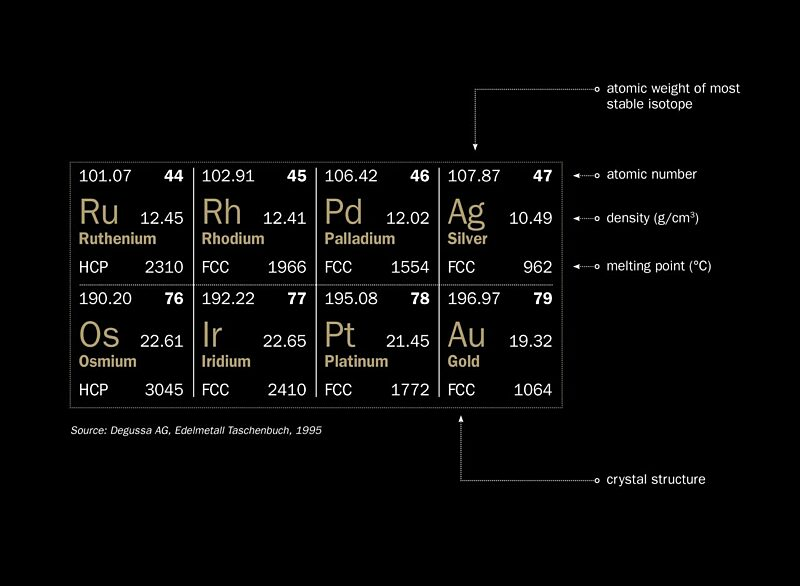# Density of GoldEnlarge

Fundamental properties of the precious metals in the periodic table.

Density is defined as the mass of a unit volume of a substance. In this article, the unit used for density is grams per cubic centimetre (g/cm3). Another term used in this branch of science is specific gravity, which is the ratio of the mass of a substance to the mass of an equal volume of water. Since water has a density of 1 g/cm3 under standard conditions, density (expressed as g/cm3) and specific gravity have the same numeric value.

It is interesting to compare the density of gold to that of the other precious metals. Gold is renowned for its high density (19.32 at room temperature, almost double that of silver) but as the table shows, some of the neighbouring platinum group metals (PGMs) are significantly denser.

The extract from the periodic table shows that in terms of atomic weight (the weight of individual atoms) gold is the heaviest precious metal. But density depends on how closely packed the atoms are in the crystal lattice. Apart from ruthenium and osmium (whose lattices are known as hexagonal close-packed, or HCP) all the precious metals have the same crystal structure, known as face-centred cubic, or FCC. The lattice cell consists of a cube with an atom at each corner and an atom at the centre of each face. For cubic lattices, the length of each cube edge is known as the lattice constant, normally measured in Ångström units (1Å=10-10m). The lattice constants for gold and silver are almost identical (at 4.078 and 4.086 respectively). Intuitively, one might have expected that the gold atoms, being almost twice as heavy as silver atoms, would result in gold having a larger lattice constant but this is not the case. This is why the density of gold is almost twice that of silver.

Similarly, the reason for gold’s neighbouring PGMs having such high densities in spite of their lower atomic weights is that their lattice constants are significantly smaller than that of gold (Ir=3.839; Pt=3.924).

Density and Temperature

It is well-known that metals expand as they get hotter and as they do, their density decreases. At just below its melting point of 1064°C, the density of gold is 18.31 (compared with 19.32 at room temperature). The density of molten gold at just above the melting point is 17.19. When molten gold solidifies there is thus a reduction in volume of 6%. This is why a shrinkage depression normally forms on the top surface of a large cast bar. With poor control of the casting process, an actual shrinkage crack can form, making the bar unacceptable for delivery in the London market.

Density and Alloying

When gold is alloyed with other metals such as silver and copper, the density of the resulting alloy is reduced. 18 carat gold (ie, with a fineness of 750) with equal proportions of silver and copper has a density of 15.4. The 9 carat gold sold in the UK typically has a density of around 11.

It is the dependence of density on alloy composition that allows the use of the Archimedes principle for detecting counterfeit or under-title gold.

Related tags